Courses

# Electronic Devices 4

## 10 Questions MCQ Test GATE ECE (Electronics) 2022 Mock Test Series | Electronic Devices 4

Description
This mock test of Electronic Devices 4 for Electronics and Communication Engineering (ECE) helps you for every Electronics and Communication Engineering (ECE) entrance exam. This contains 10 Multiple Choice Questions for Electronics and Communication Engineering (ECE) Electronic Devices 4 (mcq) to study with solutions a complete question bank. The solved questions answers in this Electronic Devices 4 quiz give you a good mix of easy questions and tough questions. Electronics and Communication Engineering (ECE) students definitely take this Electronic Devices 4 exercise for a better result in the exam. You can find other Electronic Devices 4 extra questions, long questions & short questions for Electronics and Communication Engineering (ECE) on EduRev as well by searching above.
QUESTION: 1

### For a single stage BJT common base amplifier.

Solution: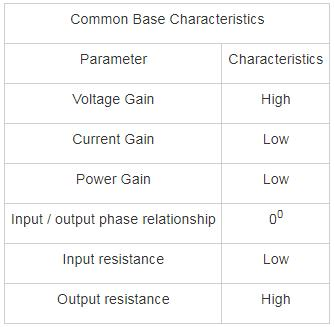∴ Voltage gain can be greater than unity but current gain is always less than unity.

*Answer can only contain numeric values
QUESTION: 2

### A bipolar transistor has an emitter current of 1 mA. The emitter injection efficiency is 0.99, base transit factor is 0.995 and depletion region recombination factor of 0.998. The base current flowing through the transistor is _____μA.

Solution:

The common emitter current gain is given by:

α = γ ∗ β ∗ δ

= 0.99 × 0.995 × 0.998 = 0.983

Collector current

Ic = αIE = 0.983 mA

Base current

IB = IE – IC

= 17 μA

*Answer can only contain numeric values
QUESTION: 3

### In a Bi-polar junction transistor the base width is 0.54 μm and base diffusion constant is DB = 25 cm2/sec. The Base transit time time is ________ × 10-10 sec

Solution:

The Base transit time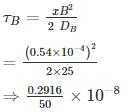⇒ 0.5832 × 10-10

*Answer can only contain numeric values
QUESTION: 4

Find the value of bias resistor (in kΩ) if quiescent collector current and voltage value are 4.6 mA and 2.2 V. The transistor has DC gain 110, VBE = 0.7 V and VCC = 4.5 V.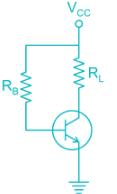Solution:

Applying KVL in collector to emitter loop

VCC = RLIC + VCE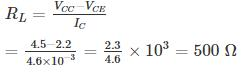Now applying KVL in collector to base loop

IBRB + VBE = VCC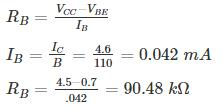QUESTION: 5

Consider two pnp bipolar junction transistors. For the first transistor when emitter to collector voltage is 5 V, VEB is 0.85 V and emitter current is 10 A. (The β for this transistor is 15). Second BJT conducts with a collector current of 1 mA and VEB = 0.70. The ratio of emitter-base junction area of the first transistor to the second transistor is ______.

(Assume KT = 26 mV)

Solution:

Given: VEC = 5V-, this means pnp transistor is operating in the active node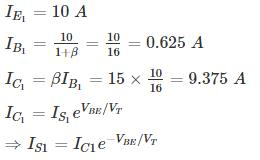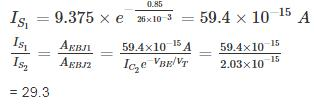*Answer can only contain numeric values
QUESTION: 6

The leakage current of a transistor with usual notation are ICEO = 410 μA, ICBO = 5 μA, and IB = 30μA. Calculate the IC ________mA

Solution:

ICEO = 410 μA, ICBO = 5 μA, IB = 30 μA

IC­ = βIB + (1 + β) ICBO

ICEO = (1 + β) ICBO

410 = (1 + β)5

β = 81

IC = 81 × 30 + 82 × 5

IC = 2.84 mA

QUESTION: 7

For what value of current gain β , the given transistor will be in saturation

(Assume Vin = 5V, VBE(SAT) = 0.8 V , VCE(SAT) = 0.2V)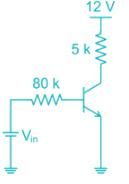Solution:

Apply kVL in base emitter loop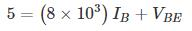at saturation VBE(sat) = 0.8

substituting the value of VBE

IB = 0.0525 mA

Apply Kvl , Form the collector to emitter

5 × 103 × I+ VCE = 12 V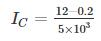= 2.36 mA

At the edge of saturation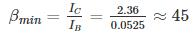Hence for β value greater than 45 the transistor will be in saturation

*Answer can only contain numeric values
QUESTION: 8

An npn bipolar transistor having uniform doping of NE = 1018 cm-3 NB = 1016 cm-3 and NC = 6 × 1015 cm-3 is operating in the inverse-active mode with VBE = -2V and VBC = 0.6 V. The geometry of transistor is shown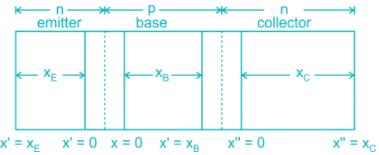The minority carrier concentration at x = xB is _____ × 1014 cm-3

(Assume n = 1.5 × 1010/cm3, Vt ­= 25 mV)

Solution: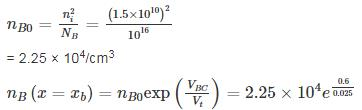= 5.96 × 1014 cm-3

QUESTION: 9

The common emitter forward current gain of the transistor shown is β = 100
The transistor is operating inSolution: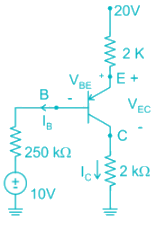Assume Transistor in Active region

20 – 2 (IB + IC) – 0.7 – 250 IB – 10 = 0

20 – 2 × 101 IB – 0.7 – 250 IB – 10 = 0

10 – 452 IB – 0.7 = 0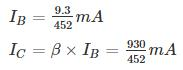20 – 2 (IB + IC) - VEC – 2IC = 0

20 – 202 IB - VEC = 0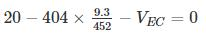VEC = 11.68 V

VCB = VCE + VEB = -VEC + VEB = - 11.68 + .7

VCB = -10.98

∴ Collector base junction is Reverse Biased

∴ Transistor is operating in forward active region

Second Method

Assume transistor in saturation region

IB min ≤ IB

i) VEB (sat) = 0.8 V

ii) VEC (sat) = 0.2 V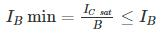IC ≠ βIB ----- Because it is valid only active region

20 – 2 (IB + IC) – 0.8 - 250 IB – 10 = 0

20 – 2 IB – 2IC – 0.8 – 250 IB – 10 = 0

10 – 252 IB – 2IC – 0.8 = 0

252 IB + 2I= 9.2      -------- (1)

20 – 2 (IB + IC) – VEC(sat) – 2IC = 0

20 – 2IB – 2IC – 0.2 – 2IC = 19.8      -------- (2)

By solving equation (1) and (2)

IB = 0.00278 mA

IC = 4.95 mA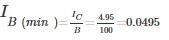∴ IB(min) > IB

∴ Transistor is operating in forward active region

QUESTION: 10

In a silicon PNP transistor the mobility of charge carries is μ4 = 1300 cm2/V-s and μp = 450 cm2/V-s and carrier life time τp = 0.10 μs. The most appropriate base width for effective transistor function is (Take T = 300° k)

Solution:

Given μn = 1300 cm2/V-s and μp = 450 cm2/V-s

Dp = 450 × 0.0259

= 11.655 cm2/s

Base diffusion length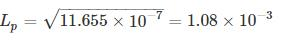= 1.08 mm

For injected holes from emitter to reach the collector they should not be recombined in base. Hence the base width should be very less compared to base diffusion length.

W ≪ LP

In all options the base width is significant compared to base diffusion length.